The Chemistry of Hydrogen

The Chemistry of Hydrogen

 Hydrides Hydrogen's Placement in the Periodic Table Properties and Formation of Hydrogen

Hydrides

Hydrogen combines with every element in the periodic table except the nonmetals in Group VIIIA (He, Ne, Ar, Kr, Xe, and Rn). Although it is often stated that more compounds contain carbon than any other element, this is not necessarily true. Most carbon compounds also contain hydrogen, and hydrogen forms compounds with virtually all the other elements as well. Compounds of hydrogen are frequently called hydrides, even though the name hydride literally describes compounds that contain an H- ion. There is a regular trend in the formula of the hydrides across a row of the periodic table, as shown in the figure below. This trend is so regular that the combining power, or valence, of an element was once defined as the number of hydrogen atoms bound to the element in its hydride.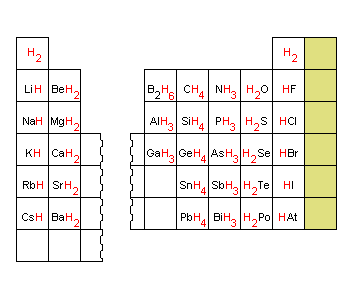Hydrogen is the only element that forms compounds in which the valence electrons are in the n = 1 shell. As a result, hydrogen can have three oxidation states, corresponding to the H+ ion, a neutral H atom, and the H- ion.

 H+ = 1s0 H = 1s1 H- = 1s2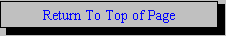Hydrogen's Placement in the Periodic Table

Because hydrogen forms compounds with oxidation numbers of both +1 and -1, many periodic tables include this element in both Group IA (with Li, Na, K, Rb, Cs, and Fr) and Group VIIA (with F, Cl, Br, I, and At).

There are many reasons for including hydrogen among the elements in Group IA. It forms compounds (such as HCl and HNO3) that are analogs of alkali metal compounds (such as NaCl and KNO3). Under conditions of very high pressure, it has the properties of a metal. (It has been argued, for example, that any hydrogen present at the center of the planet Jupiter is likely to be a metallic solid.) Finally, hydrogen combines with a handful of metals, such as scandium, titanium, chromium, nickel, or palladium, to form materials that behave as if they were alloys of two metals.

There are equally valid arguments for placing hydrogen in Group VIIA. It forms compounds (such as NaH and CaH2) that are analogs of halogen compounds (such as NaF and CaCl2). It also combines with other nonmetals to form covalent compounds (such as H2O, CH4, and NH3), the way a nonmetal should. Finally, the element is a gas at room temperature and atmospheric pressure, like other nonmetals (such as O2 and N2).

It is difficult to decide where hydrogen belongs in the periodic table because of the physical properties of the element. The first ionization energy of hydrogen (1312 kJ/mol), for example, is roughly halfway between the elements with the largest (2372 kJ/mol) and smallest (376 kJ/mol) ionization energies. Hydrogen also has an electronegativity (EN = 2.20) halfway between the extremes of the most electronegative (EN = 3.98) and least electronegative (EN = 0.7) elements. On the basis of electronegativity, it is tempting to classify hydrogen as a semimetal, as shown in the three-dimensional graph of the electronegativities of the main-group elements shown below.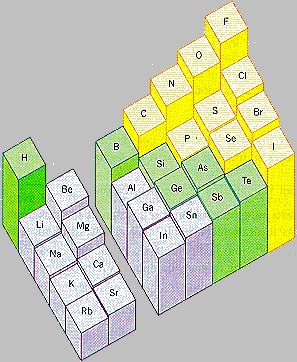This three dimensional graph of the electronegativities of the main-group elements helps us understand why it is difficult to classify hydrogen as a metal or a nonmetal.

Hydrogen is oxidized by elements that are more electronegative to form compounds in which it has an oxidation number of +1.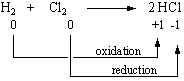Hydrogen is reduced by elements that are less electronegative to form compounds in which its oxidation number is -1.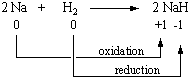Properties and Formation of Hydrogen

At room temperature, hydrogen is a colorless, odorless gas with a density only one-fourteenth the density of air. Small quantities of H2 gas can be prepared in several ways.

1. By reacting an active metal with water.

 2 Na(s) + 2 H2O(l)2 Na+(aq) + 2 OH-(aq) + H2(g)

2. By reacting a less active metal with a strong acid.

 Zn(s) + 2 HCl(aq)Zn2+(aq) + 2 Cl-(aq) + H2(g)

3. By reacting an ionic metal hydride with water.

 NaH(s) + H2O(l)Na+(aq) + OH-(aq) + H2(g)

4. By decomposing water into its elements with an electric current.

 electrolysis 2 H2O(l)2 H2(g) + O2(g)

 Practice Problem 2:Use oxidation numbers to determine what is oxidized and what is reduced in the following reactions, which are used to prepare H2 gas. (a) Mg(s) + 2 HCl(aq)Mg2+(aq) + 2 Cl-(aq) + H2(g) (b) Ca(s) + 2 H2O(l)Ca2+(aq) + 2 OH-(aq) + H2(g) Click here to check your answer to Practice Problem 2

The covalent radius of a neutral hydrogen atom is 0.0371 nm, smaller than that of any other element. Because small atoms can come very close to each other, they tend to form strong covalent bonds. As a result, the bond dissociation enthalpy for the H-H bond is relatively large (435 kJ/mol). H2 therefore tends to be unreactive at room temperature. In the presence of a spark, however, a fraction of the H2 molecules dissociate to form hydrogen atoms that are highly reactive.

 spark H2(g)2 H(g)

The heat given off when these H atoms react with O2 is enough to catalyze the dissociation of additional H2 molecules. Mixtures of H2 and O2 that are infinitely stable at room temperature therefore explode in the presence of a spark or flame.